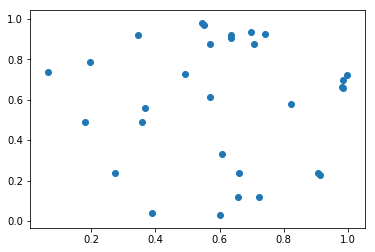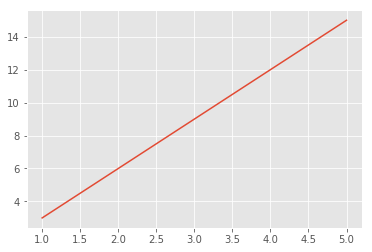In :
var1 = "Hello Jupyter Notebook!!!"
print(var1)

Hello Jupyter Notebook!!!

In :
import pandas as pd
pd.DataFrame([[1,"abc"],[2,"xyz"],[3,"123"],[4,"1a2b"]], columns=("ID","Name"))

Out:
ID Name
0 1 abc
1 2 xyz
2 3 123
3 4 1a2b
In :
%matplotlib inline

import numpy as np
import matplotlib.pyplot as plt

N = 30
x = np.random.rand(N)
y = np.random.rand(N)

plt.scatter(x,y)
plt.show()In :
%matplotlib inline

import numpy as np
import matplotlib.pyplot as plt

plt.style.use("ggplot")

x = [1, 2, 3, 4, 5]
y = [3, 6, 9, 12, 15]
plt.plot(x,y)

Out:
[<matplotlib.lines.Line2D at 0xaa97660c>]